Get instant live expert help with Excel or Google Sheets“My Excelchat expert helped me in less than 20 minutes, saving me what would have been 5 hours of work!”

#### Post your problem and you’ll get expert help in seconds.

Your message must be at least 40 characters
Our professional experts are available now. Your privacy is guaranteed.

# How to Make a Cell Constant in Excel – Excelchat

We can keep a formula constant with the F4 key. In this tutorial, we will learn the easy way of keeping the value of a cell constant.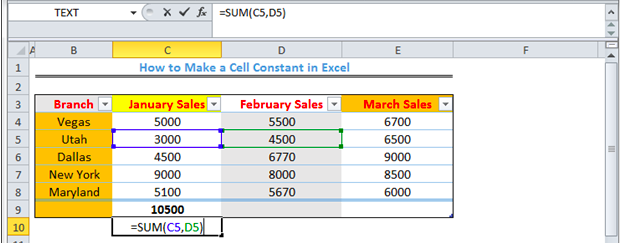Figure 1 – How to keep value constant in formula

## How to Keep a Cell Constant

• We will use figure 2 to illustrate how we can keep a formula constant regardless of where we copy the formula.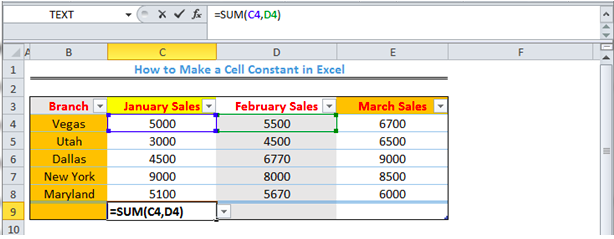Figure 2 – How to keep a reference constant

• When we press enter for the formula in figure 2, we will get the result. If we copy down the formula in Cell C9, the cell reference changes to sum Cell C5 and Cell D5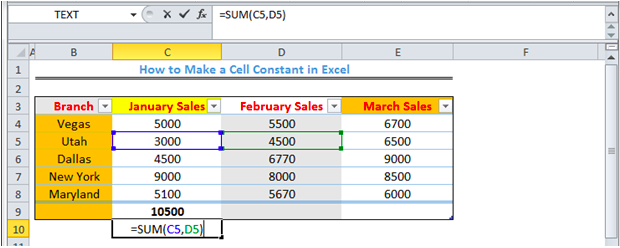Figure 3 – How to keep a value constant

## How to Keep Cell Value Constant with the F4 key

• Assuming we intend to sum the values in Column C while keeping the “value” in Column D as D4 in the formula, we will add the dollar sign before copying down the formula as shown in Figure 4. We can also press the F4 Key when we take our cursor to the positions before the Column Letter (D) and the Column Number (4).

Formula `=SUM(C4,\$D4)`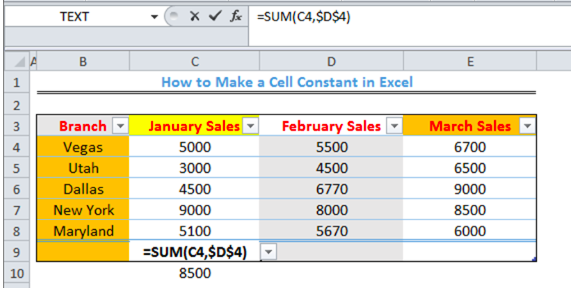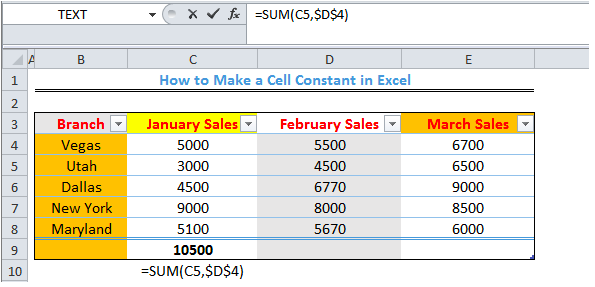Figure 4 – How to keep value constant formula

Note – If we have a formula like =SUM(\$C\$4,\$D\$4), that will tell Excel to maintain the same cell reference (the formula), no matter the cell it is copied to.

## Instant Connection to an Excel Expert

Most of the time, the problem you will need to solve will be more complex than a simple application of a formula or function. If you want to save hours of research and frustration, try our live Excelchat service! Our Excel Experts are available 24/7 to answer any Excel question you may have. We guarantee a connection within 30 seconds and a customized solution within 20 minutes.